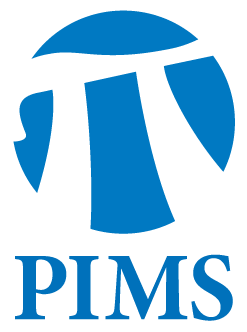## Special Geometry Seminar: Laurent Meersseman

• Date: 07/06/2012
Lecturer(s):
Laurent Meersseman, Université de Bourgogne & Centre de Recerca Matematica, Universitat Autonoma de Barcelona
Location:

University of British Columbia

Topic:

"Polarized CR-structures"

Description:

Let $X=(X^{diff}, J)$ be a compact complex manifold. Kodaira and Spencer developed from 1957 a theory to address the problem: describe the set $I$ of complex structures on $X^{diff}$ close to $J$. The crowning piece of this theory is Kuranishi's Theorem (1962) which states that the (infinite-dimensional) analytic set $I$ is isomorphic to the product of a (finite-dimensional) analytic set $K$ by a vector space. Moreover, every structure of $I$ is isomorphic to one of $K$, so that $K$ contains all classes of isomorphisms of complex structures close to $J$.

Let $(E,J)$ be a CR-structure on a smooth manifold $X^{diff}$. It is natural to ask the same question in that context that is: describe the set $I$ of CR-structures close to $(E,J)$. However, this is out of reach for a general $(E,J)$.

In this talk, I will introduce the notion of polarized CR-structures, which are a very special type of CR-structures; and give a description of the set $I$ in the case where $(E,J)$ is polarized.

Schedule:

3:00pm in WMAX 110.  Coffee and cookies to be served at 2:45pm.